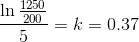Scan QR code or get instant email to install app

Question:

# A colony of 200 bacterial cells is placed in a petri dish. After 5 hours, the bacteriologist finds 1,250 bacterial cells in the dish and assumes that the bacteria are growing at an exponential rate. In such a case, what is the growth constant associated with this colony of cells?

A 0.37
explanation

1212Use the general form of the exponential equation to represent the bacterial growth: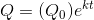, where Q is the population at time t,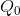is the initial population, and k is the growth constant.
Substitute the given values into the equation and solve for k: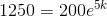, which gives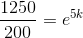, and taking the natural log of both sides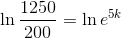, and isolating k